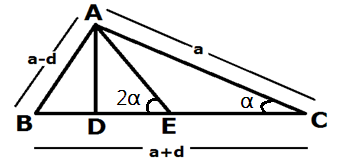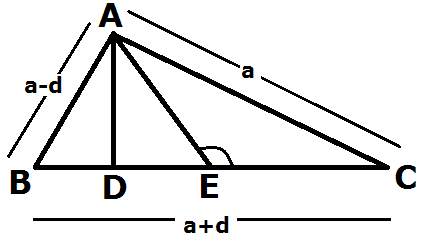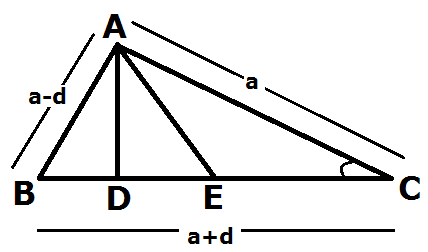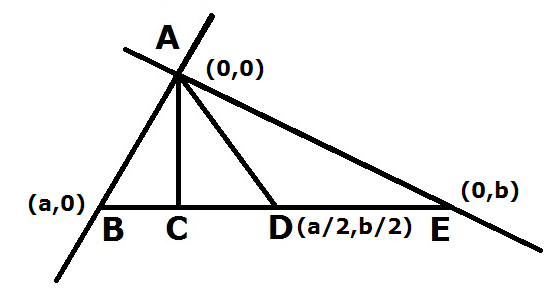PiyushTheorem

PiyushTheorem: In a Right-Angled Triangle with sides in A.P. Series, the distance between the point of intersection of median & altitude at the base is 1/10 Th the sum of other two sides. This Theorem applies in Two Conditions:

1.The Triangle must be Right-Angled.

2.Its Sides are in A.P. Series.

1.Proof with TrigonometryTan  α   =AD/DC AD= DC Tan  α   —————–1 Tan  α = AD/DE AD= DE Tan2 α   —————-2 DC Tan  α = DE Tan 2 α (DE+EC)  Tan  α = DE Tan 2 α DE Tan  α  + EC Tan  α = DE Tan 2 α DE Tan  α  + EC Tan  α = 2 DE Tan  α / (1- Tan2  α   ) DE Tan  α   – DE Tan3  α + EC Tan  α –EC Tan3  α  = 2DE Tan  α EC Tan  α –EC Tan3  α – DE Tan3  α = 2DE Tan  α – DE Tan  α Tan  α (EC – EC Tan2  α – DE T an2  α )= DE Tan  α DE Tan2  α  – DE = EC Tan2  α  – EC -DE ( Tan2  α + 1) = -EC (1 – Tan2  α ) DE (sin2 α  /cos2 α + 1) = EC (1- sin2 α  /cos2 α ) DE (sin2 α + cos2 α /cos2 α ) = EC (cos2 α – sin2 α /cos2 α ) DE (sin2 α  + cos2 α ) = EC(cos2 α  –sin2 α ) DE (sin2 α  + cos2 α ) = EC (cos2 α  –sin2 α )           where (sin2 α  + cos2 α =1) & (cos2 α  –sin2 α = cos2 α  )              DE= EC cos2 α   cos α   =a/a+d   & sin α = (a-d)/ (a +d) cos2 α  = a2/ (a +b) 2 sin2 α  = (a-d) 2/ (a+ d) 2 DE= EC (cos2 α    – sin2 α ) = EC (a2 / (a +b) 2 – (a-d) 2/ (a +d) 2 = EC (a2 – (a-d) 2/ (a +d) 2 = EC (a –a +d) (a+ a-d)/ (a+ d) 2 = EC (d) (2a -d)/ (a+ d) 2 = (a +d)/2(d) (2a -d)/ (a +d) 2 ————- where EC= (a +d)/2 = (d) (2a -d)/2(a +d) = (d) (8d -d)/2(4d+d)       ——————where a= 4d (as per the Theorem) = 7d2 /2(5d) = 7d /10 = (3d+4d)/10= (AB+AC)/10

2.Proof with Obtuse Triangle TheoremAC2=EC2 +AE2 +2CE.DE       where EC = (  a +d) /2,AE=( a +d)/2 a2 = (a +d/2)2 + (a+ d/2)2 + 2(a +d)/2DE = (a +d/2) (a+d+2DE) = (a +d/2) (a+d+2DE)   where a=4d 16d2 = (5d/2) (5d+2DE) 32d/5 = 5d + 2DE 32d/5 – 5d = 2DE 32d -25d/5 = 2DE DE =7d/10 = (3d+4d)/10 = (AB+AC)/10

3.Proof with Acute Triangle TheoremAB2= AC2+BC2 – 2BC.DC (a-d) 2= a2 + (a+ d) 2 -2(a+ d) (DE+EC)         where AB= (a-d), AC=a, BC =( a +d) & EC= (a +d)/2 (a-d) 2 – (a +d)2 = a2  -2(a +d)(DE+EC) (a- d –a-d) (a -d +a +d)  = a2 -2(a+ d) (2DE+a+d)/2 2(-2d) (2a) = 2a2 -2(a +d) (2DE+a+d) -8ad – 2a2 = -2(a +d) (2DE+a+d) -2a (4d   +a) = -2(a +d) (2DE+a+d) a (4d  + a) = (a +d)(2DE+a+d) 4d (4 d + 4d) = (4d+d) (2DE+4d+d) 4d (8d) = (5d) (2DE+5d) 32d2/5d =   (2DE+5d) 32d/5 =   (2DE+5d) 32d/5 – 5d =   2DE (32d – 25d)/5 =   2 DE DE = 7d/10 = (3d+4d)/10 = (AB+AC)/10

4. Proof with Co-ordinates GeometryEquation of BE Y – 0 =b-0/0-a(X – a) Y = -b/a(X) + b——————- (1) M1 = -b/a For perpendicular M1M2= -1 So M2=a/b Equation of AC Y – 0 = a/b(X-0) Y=a/b(X) —————— (2) Put Y value in equation (1) a/b(X) + b/a(X) =b X (a2+b2/a b) = b X = ab2/ (a2 + b2) To get Value of Y, put X value in equation (2) Y = a/b (ab2/ (a2+b2) Y = a2b/ (a2+b2) Here we got co-ordinates of Point C – ab2/ (a2 + b2), a2b/ (a2+b2) and co-ordinates of point d is (a/2, b/2) because d is midpoint. As per the “Theorem” a=z-d, b=z, c = z+ d (z +d) 2= (z-d) 2+z2 from here z=4d so a=3d and b=4d Put value of a & b ab2/ (a2 + b2), a2b/ (a2+b2) & (a/2, b/2) ab2/ (a2 + b2) = 48d/25 a2b/ (a2+b2) = 36d/25 a/ 2=3d/2 b/ 2 =4d/2 CD2= (48d/25 -3d/2)2-(36d/25-4d/2)2 = (96d-75d/50)2 + (72d-100d/50)2 = (21d/50)2 + (-28d/50)2 = (441d2/2500) + (784d2/2500) = (1225d2/2500) CD= 35d/50 = 7d/10 = 7d/10 = (3d+4d)/10 = (AB+AE)/10

https://piyushtheorem.wordpress.com/2017/02/08/a-theorem-on-right-angled-triangles/Himesh Bhai Creator

(No description available)

Suggested Creators